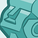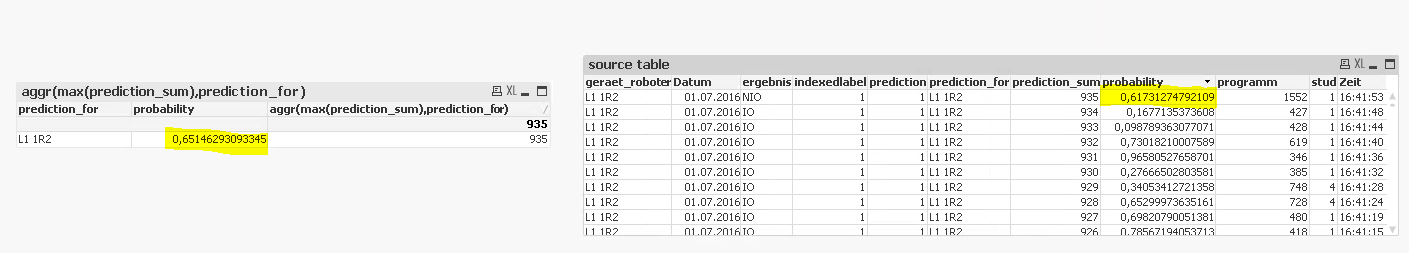# QlikView App Dev

Discussion Board for collaboration related to QlikView App Development.

Announcements
Skip the ticket, Chat with Qlik Support instead for instant assistance.
cancel
Showing results for
Did you mean:Contributor

## Show record with max field value using aggregation

Hello Experts,

I have situation: I want to find record with max value of field "prediction sum". I am using the aggregation: aggr(max(prediction_sum),prediction_for). Field "prediction_for" has one value across the table. After aggregation I have one record, but value of the field "probability" is different than in source table. Why? (pic below)1 Solution

Accepted SolutionsMVP

Use calculated dimension like:

=Aggr(FirstSortedValue( probability,  -prediction_sum), prediction_for)

6 RepliesMVP

"probability" is a field or you are using some expression to create it?MVP

May be

FirstSortedValue(probability, aggr(max(prediction_sum),prediction_for)Contributor
AuthorMVP

FirstSortedValue( Aggr(probability, prediction_for), -aggr(prediction_sum, prediction_for))

if this doesn't work, try to share your sample qvw.Contributor
Author

In attachment is an example qvw. I thought that when I use aggregation it will show the one record with max field and other fields related to this value. Something is missing?MVP

Use calculated dimension like:

=Aggr(FirstSortedValue( probability,  -prediction_sum), prediction_for)Community Browser